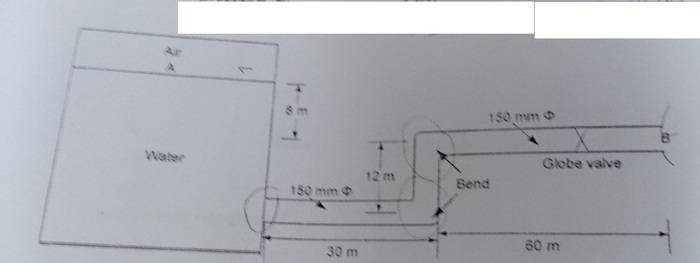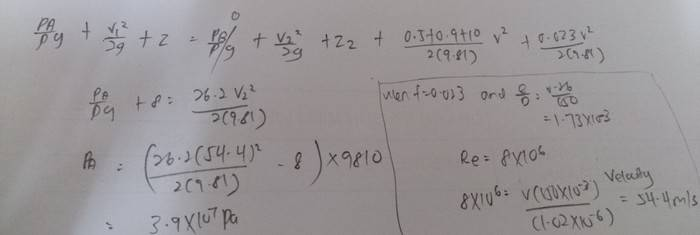# Find velocity in pipe

• foo9008

## Homework Statement

water flows from flow tank at A through cat iron pipeline to free surface at B . Given friction factor , f = 0.023, kinematic viscosity = 1.02 x10^-6 (m^2 0 /s , coefficient of losses for entrance , bend , valve = 0.5 , 0.9 and 10 . [/B]
in this question , i am asked to find the velocity , flow rate , type of flow and pressure at A .
for the first part , I'm asked to find the velocity . The flow rate is not given , how could the velocity be found ? Since Q = Av ,## The Attempt at a Solution

i assume the flow as laminar at first , where reynold number less than 2000 ,
so i have f = 64 / Re
0.023 = 64(1.02 x10^-6)/ 1000 / ( 1000 x v x 150x10^-3) , v = 2.08x10^-8 , is my answer correct ? my reynold number is rather samll , which is 3.05 only , is it possible ? [/B]

## Homework Statement

water flows from flow tank at A through cat iron pipeline to free surface at B . Given friction factor , f = 0.023, kinematic viscosity = 1.02 x10^-6 (m^2 0 /s , coefficient of losses for entrance , bend , valve = 0.5 , 0.9 and 10 . [/B]
in this question , i am asked to find the velocity , flow rate , type of flow and pressure at A .
for the first part , I'm asked to find the velocity . The flow rate is not given , how could the velocity be found ? Since Q = Av ,## The Attempt at a Solution

i assume the flow as laminar at first , where reynold number less than 2000 ,
so i have f = 64 / Re
0.023 = 64(1.02 x10^-6)/ 1000 / ( 1000 x v x 150x10^-3) , v = 2.08x10^-8 , is my answer correct ? my reynold number is rather samll , which is 3.05 only , is it possible ? [/B]

You're given a friction factor for the cast iron pipe of f = 0.023, which appears to be for fully turbulent flow. This would suggest that the initial assumption of laminar flow would be incorrect.

Reynolds No. for the flow in the pipe is going to be calculated by the formula ##Re = V * D / \nu##,
or Re = 147060 * V, where V is the velocity of the flow in m/s.

Your calculated flow velocity is 2 × 10-8 m/s, which means the water would take 161 years to move from the entrance of the pipe to the exit, so no, you need to try again.

BTW, laminar flow where water is the fluid realistically occurs only where the flow velocity is extremely slow, as you have already found, or the diameter of the pipe is extremely small.

For a fluid to have laminar flow in a 150 mm pipe, its kinematic viscosity must be much, much greater than the viscosity of water.

•foo9008
You're given a friction factor for the cast iron pipe of f = 0.023, which appears to be for fully turbulent flow. This would suggest that the initial assumption of laminar flow would be incorrect.

Reynolds No. for the flow in the pipe is going to be calculated by the formula ##Re = V * D / \nu##,
or Re = 147060 * V, where V is the velocity of the flow in m/s.

Your calculated flow velocity is 2 × 10-8 m/s, which means the water would take 161 years to move from the entrance of the pipe to the exit, so no, you need to try again.

BTW, laminar flow where water is the fluid realistically occurs only where the flow velocity is extremely slow, as you have already found, or the diameter of the pipe is extremely small.

For a fluid to have laminar flow in a 150 mm pipe, its kinematic viscosity must be much, much greater than the viscosity of water.
sorry , i made a mistake . i forgot it's kinematic viscosity , in my previous working , i assume the kinematic viscosity as dynamic viscosity ...

pls ignore my previous working.

so, how to find the velocity in this question ? the head loss and flow rate are not given... if it's turbulent the equation that i can use is hf (head loss) = fL(v^2) / 2gD

in the previous working , i use 32(dynamic viscosity)(L)(veloxity) / (rho)(g)(D^2) which is only applicable for laminar flow...

can anyone help ?

Bump

sorry , i made a mistake . i forgot it's kinematic viscosity , in my previous working , i assume the kinematic viscosity as dynamic viscosity ...

pls ignore my previous working.

so, how to find the velocity in this question ? the head loss and flow rate are not given... if it's turbulent the equation that i can use is hf (head loss) = fL(v^2) / 2gD

in the previous working , i use 32(dynamic viscosity)(L)(veloxity) / (rho)(g)(D^2) which is only applicable for laminar flow...

can anyone help ?
Well, you have to do a bit of analysis here.

You can write a modified Bernoulli equation which includes the head loss due to friction, according to the Darcy relation, which should give you a relationship based on flow velocity. The continuity equation can also be used to relate flow velocity into the pipe with flow velocity out of the pipe.
sorry , i made a mistake . i forgot it's kinematic viscosity , in my previous working , i assume the kinematic viscosity as dynamic viscosity ...

pls ignore my previous working.

so, how to find the velocity in this question ? the head loss and flow rate are not given... if it's turbulent the equation that i can use is hf (head loss) = fL(v^2) / 2gD

in the previous working , i use 32(dynamic viscosity)(L)(veloxity) / (rho)(g)(D^2) which is only applicable for laminar flow...

can anyone help ?
Well, start by figuring out how fast the water must flow inside a 150 mm cast iron pipe to become fully turbulent.

Well, you have to do a bit of analysis here.

You can write a modified Bernoulli equation which includes the head loss due to friction, according to the Darcy relation, which should give you a relationship based on flow velocity. The continuity equation can also be used to relate flow velocity into the pipe with flow velocity out of the pipe.

Well, start by figuring out how fast the water must flow inside a 150 mm cast iron pipe to become fully turbulent.

when f = 0.023, and e/D = 0.026 / 150 , i gt Re= 8x10^6 , does it make sense?

so , i gt v = 54.4m/s , is it okay ?

however the pressure that i found is very big , which is 3.9x1067 pa , is it correct ?

The pressure act on exit of B is atmospheric pressure, I took it as 0...Last edited:
when f = 0.023, and e/D = 0.026 / 150 , i gt Re= 8x10^6 , does it make sense?

so , i gt v = 54.4m/s , is it okay ?

however the pressure that i found is very big , which is 3.9x1067 pa , is it correct ?

The pressure act on exit of B is atmospheric pressure, I took it as 0...Your flow Reynolds No. and resulting flow velocity look high for fully turbulent flow for this size pipe. Did you read your Moody diagram correctly?

Remember, head loss thru straight pipe is calculated as f * (L/D) * v2 / (2g).

Fixing these calculations will result in a different pressure at point A. Always double check what you write. You have Pa = 3.9 × 1067 Pa in your post above. The image has 3.9 × 107 Pa.

Remember to calculate the flow rate once you have the flow velocity.